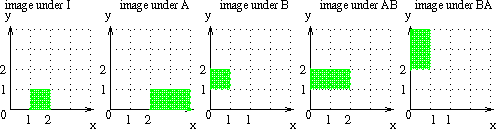5: Transformations and Matrix Multiplication

Some Transformations of R2

Section 4 was about how transformations can arise.
Now we're going to look at some basic examples of linear transformations, and see how they are represented by matrices.
Remember the important fact the the rows of the transformation matrix give the linear equations of the transformation, and the columns give the images of (1,0), and (0,1). The first column of the 2 by 2 matrix representing the transformation is the image of (1,0), and the second is the image of (0,1).
We'll See:
• Dilations
• Contractions
• Stretching
• Shears
• Rotations
• Projection

Then we'll go on to look at linear combinations of these, that is, composition of linear transformations.

Dilations and Contractions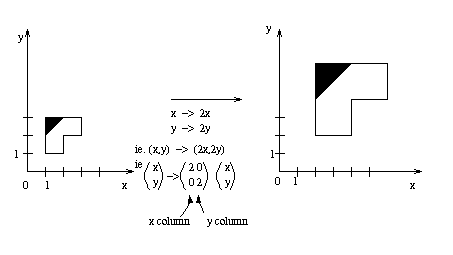The matrix which scales by 2 in three dimensions is given by:
 2 0 0 0 2 0 0 0 2
By 3 In 7 dimensions:
 3 0 0 0 0 0 0 0 3 0 0 0 0 0 0 0 3 0 0 0 0 0 0 0 3 0 0 0 0 0 0 0 3 0 0 0 0 0 0 0 3 0 0 0 0 0 0 0 3
(You have to imagine brackets round these for them to really be matrices.)
The seven dimensional transformation might correspond to multpilying the ingredients for a recipie with 7 ingredients by three, since it's only supposed to be for 3 people, but nine will come round for tea.
If you use a number smaller than one, instead of 2 or three above, you get a contraction instead of a dilation.

Shears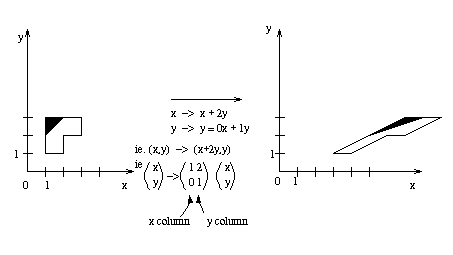Above is a shear of amount s in the x direction. We'll see some more shears in class. I drew the image of the grid to help me find the image of the shape: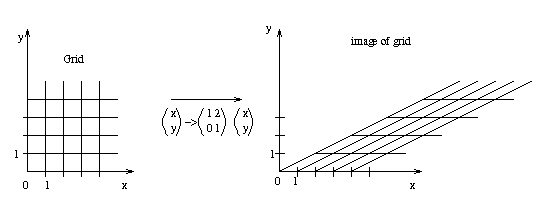Stretch

The following is a stretch.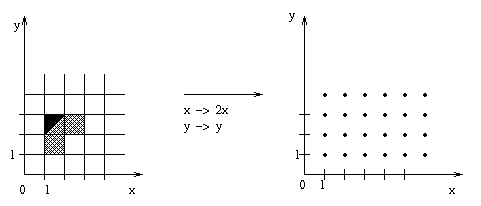Exercise: Write down the matrix, and draw in the image of the grid, finding the images of (1,0), and (0,1) first. Use that to draw the image of the object. (The dots are a guide line, marking points distance 1 apart in vertical and horizontal direction.)

Rotation

Excercise for a rotation about the origin of 45 degrees anti-clockwise: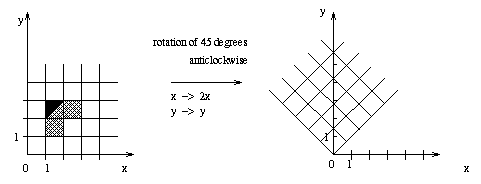In the diagram below, the image of the grid is drawn.
Questions:
• What are the images of (1,0) and (0,1)?
• What is the matric of the transformation?
• What is the image of the object?
(We will do this in class, and other rotations, and also reflections.)

How to Compose Transformations?

What happens if we do a shear then a rotation? When we make one transformation, and then another, this is called composing transformations.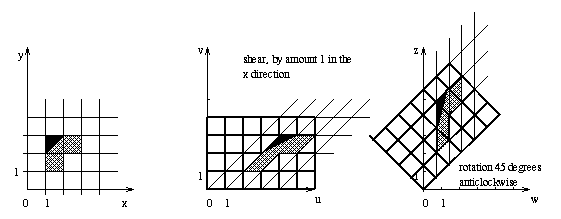The matrix for the shear is
 / 1 1 \ \ 0 1 /

The matrix for the rotation is
 / s -s \ \ s s /
(s stands for the square root of 1/2.)
How can we describe the composition?
(x,y)=(1,0) has ended up at (z,w)=(s,s), and (0,1) has ended up at (0,2s), (where s is the square root of 1/2).
This tells us the columns of the matrix, so we know that the matrix will be
 / s 0 \ \ s 2s /

Composition of tranformations and Matrix Multplication

The composition of transformations is represented by the composotion of matrices. Eg, to represent that the shear followed by the rotation is the third matrix, we write:
 / s -s \ / 1 1 \ = / s 0 \ \ s s / \ 0 1 / \ s 2s /

This is called matrix multiplication. Notice that the matrices multiply from right to left, that is, the first one is right most. This makes sence because of the way we wrote the vector on the right of the matrix in matrix and vector multiplication.
There is a general rule for matrix multiplication:
 / a b \ / e f \ = / ae+bg af+bh \ \ c d / \ g h / \ ce+dg cf+dh /

Check it and see this rule works for the above example. We'll see in the lectures why this rule works, and next we'll look at matrices of larger sizes.
Note, in class someone asked about is the inverse of the matrix of a rotation was the same as it's transpose. The answer is yes, and we can check this out in a couple of different ways.

Matrix Multiplication

We've seen how to compose two linear transformations in two variables, and how this corresponds to multiplying two by two matrices.
Now we'll define matrix multiplication more generally.
Recall how a matrix and vector can be multiplied, eg:
 / 1 2 3 4 \ / x \ / x + 2y + 3z + 4w \ | 5 6 7 8 | | y | = | 5x + 6y + 7z + 8w | | 0 1 0 2 | | z | | 0x + y + 0z + 2w | \ 1 1 -1 1 / \ w / \ x + y - z + w /

For two matrices:
 / 1 2 \ / x z \ \ 3 4 / \ y w /

We can think of the second matrix like two columns, do the matrix vector multiplication, and combine the results:
 / 1 2 \ / x \ = / x+2y \ \ 3 4 / \ y / \ 3x+4y /

 / 1 2 \ / z \ = / z+2w \ \ 3 4 / \ w / \ 3z+4w /

So:
 / 1 2 \ / x z \ / x+2y z+2w \ \ 3 4 / \ y w / \ 3x+4y 3z+4w /

And in this process, we can think of the first matrix being split into two row. Each row of the first matrix combines with each column of the second matrix.
Generally: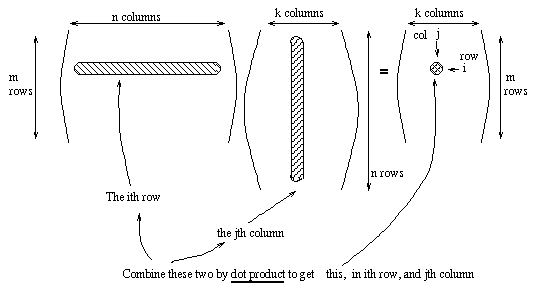Dot product is defined below. Note, the dot product is often called the inner product (I like the word "dot" better personally, but the book likes "inner".)

The inner product (Also called "dot product")

We might have one vector that gives the amount of some things, like the recipie vectors in lecture 2, and some other vectors might give the prices, or callorie content, or some thing else. The dot product will tell you the total price or total callorie content, or whatever. Eg. the following tables give you amounts of ingredients of some recipies, and the average price in different countries. (Warning: These recipes have not been tested.)
 ingredients eggs flour 1 2 3 4 1 2 3 4 0 7 5 2 5 2 1 1 2 3 0 0 1 1 2 3 4 2 3 2 1 1 1 1

So, if you make recipe 1 in country B, it will cost:
(1,2,3,4).(4,2,3,2)=1*4+2*2+3*3+4*2=25

The matrix you get by multiplying the amount matrix and the price matrix will give you a table of all possible prices of the different recipes in different countries. We have to turn the second matrix on it's side for this to work:
 / 1 2 3 4 \ / 1 4 1 \ | 1 2 3 4 | | 1 2 1 | | 0 7 5 2 | | 2 3 1 | | 5 2 1 1 | \ 3 2 1 / \ 2 3 0 0 /

Geometrical interpretation of Inner Product (or dot product)

This is not quite the same as addition or multiplication by a scalar, since when we take the dot product, we don't get another vector, we get a number.
Example: What are
• (1,0).(0,1)?
• (1,0).(1,1)?
• (1,0).(1,2)?
• (1,0).(2,2)?
• (1,0).(2,1)?

If we write the number (1,0).(a,b) next to the point (a,b), we get something like this: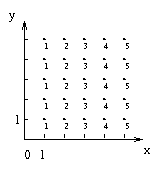This gives us the projection of (a,b) onto (1,0), ie, the amount of (a,b) in the direction (1,0).
We'll go over the projection in more detail tomorrow.

Inverse of transformations

Geometrically, the inverse of a transformation means what transformation can you apply to get back to the original situation. Eg, if you rotate something 30 degrees anticlockwise, then to get it back to where it was, you must rotate it 30 degrees clockwise.
What are the inverse transformations of the following transformations? Describe in words.
• Rotation 50 degrees clockwise
• Dilation by a factor of 2
• A reflection in a line at 30 degrees to the x-axis
• A shear, amount 2 in the x-direction
• A stretch by amount 5 in the y direction

Next question: write matrices for all the above transformations, and for their inverses.
How can we find the inverse of other transformations? Eg, what is the inverse of
 / 3 1 \ \ 2 5 /

How can we find an inverse? Can we even be sure there is an inverse? Can you think of some transformations where theres no way we'll be able to recover the original position?
In the class we'll look at the geometry of the above transformation, and show how to find the inverse by using row reduction.
The row reduction method we'll look at will work well in all dimensions, but there is also a formuala for 2 dimensional transformations:
The inverse of
 / a b \ \ c d /
is
 1/(ad-bc) / d -b \ \ -c a /

Check that it works!
We'll use this formular in some examples.

Assignment

This is to be handed in to be marked on Tuesday 26th May.
• From section 2.7, questions: 4, 8
• From section 3.1, questions: 16, 19, 23, 33
• From section 3.2, questions: 12, 13, 14, 15, 32

Also answer and hand in the following:
If the Matrix A is given by each of the following in turn:
 / 1 0 \ / -1 0 \ / 0 1 \ / 1 1 \ / 0 1 \ \ 1 0 / \ 0 2 / \ -1 0 / \ 0 1 / \ 0 0 /
Then
• What is A6? (The sixth power of A)
• What do you think AN looks like for very large N? (ie, what is the limit as N tends to infinity?)
• Can you give geometrical interpretations for these matrices and their powers?

What will be on the quiz

On Thursday 21st of May there will be a quiz, with a question like the following:
• What is the 2 by 2 matrix A which gives a stretch of amount 2 in the x direction?
• What is the 2 by 2 matrix B which gives a reflection in the line x=y?
• What is AB? (matrix of reflection followed by stretch)
• What is BA? (matrix of stretch followed by rotation)
• On the graphs below, draw the images of the square with vertices at (1,0), (2,0), (1,1), (2,1), under I (the identity), A, B, AB, and BA.
• Write down the inverse matrices of A, B, AB, and BA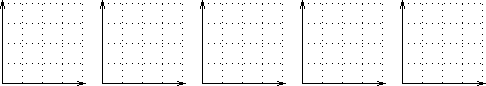The way to find what the matrices are for A and B is to think about what happens, work out what the images of (1,0) and (0,1) will be, and make the first column the image of (1,0), and the second column the image of (0,1).
A stretch of amount 2 in the x direction will make (1,0) go to (2,0), but (0,1) will not change, since it's zero distance in the x direction, so zero times zero is still zero. So the matrix A is:
 / 2 0 \ \ 0 1 /

A reflection in the line x=y will make (1,0) go to (0,1), and (0,1) will go to (1,0), so the matrix B is:
 / 0 1 \ \ 1 0 /

Use the rules for matrix multiplication to find the products:
 / 2 0 \ / 0 1 \ = / 0 2 \ \ 0 1 / \ 1 0 / \ 1 0 /
 / 0 1 \ / 2 0 \ = / 0 1 \ \ 1 0 / \ 0 1 / \ 2 0 /

For finding the images of A and B, this can be done just be considering the geometric definitions. For AB and BA, there are two methods, either work out geometrically, remembering that AB means do B first, and then A, or else algebraically, eg, we found AB above, so we can do the calculations to find out where (1,0), (2,0), (1,1), (2,1) get sent to:
 / 0 2 \ / 1 \ = / 0 \ \ 1 0 / \ 0 / \ 1 /
 / 0 2 \ / 2 \ = / 0 \ \ 1 0 / \ 0 / \ 2 /
 / 0 2 \ / 1 \ = / 2 \ \ 1 0 / \ 1 / \ 1 /
 / 0 2 \ / 2 \ = / 2 \ \ 1 0 / \ 1 / \ 2 /

The caluculation for BA can be made in a similar way.
Then we can draw the images on the graphs: